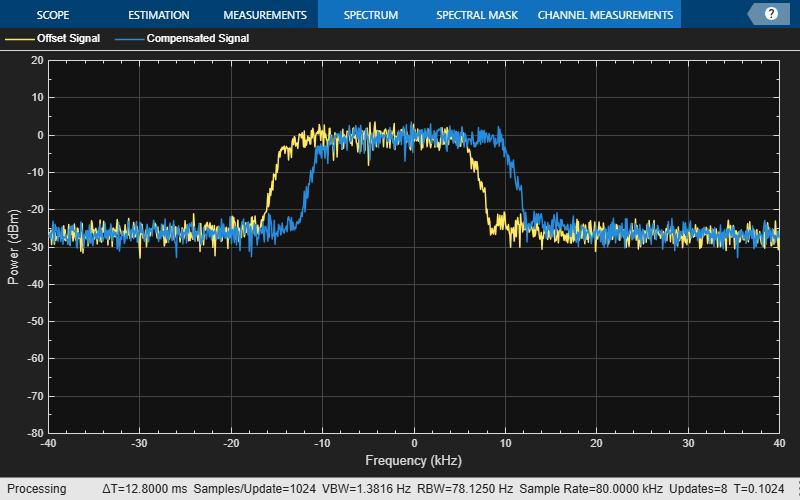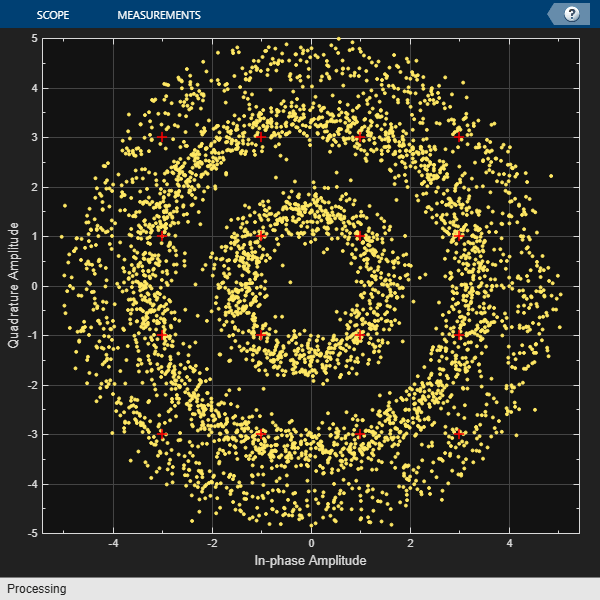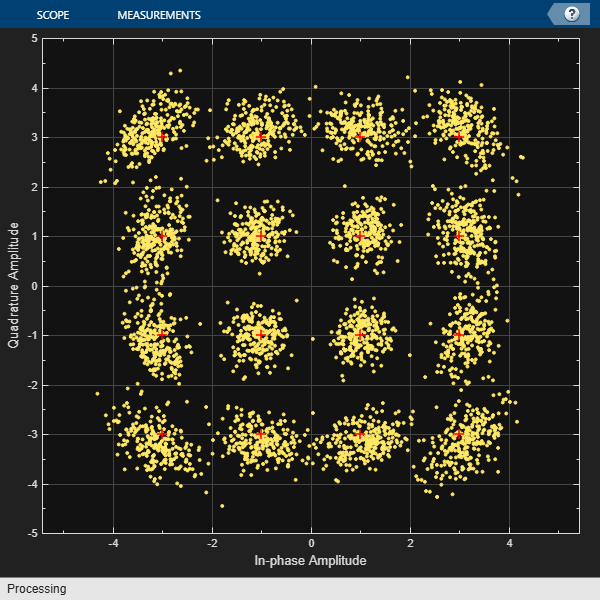# comm.CoarseFrequencyCompensator

Compensate for frequency offset of PAM, PSK, or QAM signal

## Description

The `comm.CoarseFrequencyCompensator` System object™ compensates for the frequency offset of received signals using an open-loop technique.

To compensate for the frequency offset of a PAM, PSK, or QAM signal:

1. Create the `comm.CoarseFrequencyCompensator` object and set its properties.

2. Call the object with arguments, as if it were a function.

## Creation

### Syntax

``coarseFreqComp = comm.CoarseFrequencyCompensator``
``coarseFreqComp = comm.CoarseFrequencyCompensator(Name,Value)``

### Description

example

````coarseFreqComp = comm.CoarseFrequencyCompensator` creates a coarse frequency offset compensator System object. This object uses an open-loop technique to estimate and compensate for the carrier frequency offset in a received signal. For more information about the estimation algorithm options, see Algorithms.`coarseFreqComp = comm.CoarseFrequencyCompensator(Name,Value)` specifies properties using one or more name-value arguments. For example, `Modulation='QPSK'` specifies quadrature phase-shift keying modulation.```

## Properties

expand all

Unless otherwise indicated, properties are nontunable, which means you cannot change their values after calling the object. Objects lock when you call them, and the `release` function unlocks them.

If a property is tunable, you can change its value at any time.

Modulation type, specified as one of,

• `'BPSK'` – Binary phase shift keying

• `'QPSK'` – Quadrature phase shift keying

• `'OQPSK'` – Offset quadrature phase shift keying

• `'8PSK'` – 8-phase shift keying

• `'PAM'` – Pulse amplitude modulation

• `'QAM'` – Quadrature amplitude modulation

Data Types: `char` | `string`

Algorithm used to estimate the frequency offset, specified as `'FFT-based'` or `'Correlation-based'`.

#### Dependency

To enable this property, set `Modulation` to `'BPSK'`, `'QPSK'`, `'8PSK'`, or `'PAM'`. This table shows the valid combinations of the modulation type and the estimation algorithm.

ModulationFFT-Based AlgorithmCorrelation-Based Algorithm
`BPSK`, `QPSK`, `8PSK`, `PAM`YesYes
`OQPSK`, `QAM`YesNo

Use the correlation-based algorithm for HDL implementations and for other situations in which you want to avoid using an FFT.

Data Types: `char` | `string`

Frequency resolution for the offset frequency estimation in hertz, specified as a positive scalar. This property establishes the FFT length used to perform spectral analysis and must be less than the sample rate.

Data Types: `double`

Maximum measurable frequency offset in hertz, specified as a positive scalar.

The value of this property must be less than fsamp / M. For more details, see Correlation-Based Estimation.

#### Dependency

To enable this property, set the `Algorithm` property to `'Correlation-based'`.

Data Types: `double`

Sample rate in samples per second, specified as a positive scalar.

Data Types: `double`

Samples per symbol, specified as an even positive integer greater than or equal to `4`.

#### Dependency

To enable this property, set `Modulation` to `'OQPSK'`.

## Usage

### Syntax

``y = coarseFreqComp(x)``
``[y,estimate] = coarseFreqComp(x)``

### Description

example

````y = coarseFreqComp(x)` returns a signal that compensates for the carrier frequency offset of the input signal.```

example

````[y,estimate] = coarseFreqComp(x)` returns a scalar estimate of the frequency offset.```

### Input Arguments

expand all

Input signal, specified as a column vector.

Data Types: `single` | `double`

### Output Arguments

expand all

Compensated output signal, returned as a complex column vector with the same dimensions and data type as the input `x`.

Estimate of the frequency offset, returned as a scalar.

## Object Functions

To use an object function, specify the System object as the first input argument. For example, to release system resources of a System object named `obj`, use this syntax:

`release(obj)`

expand all

 `info` Characteristic information about coarse frequency compensator `clone` Create duplicate System object `isLocked` Determine if System object is in use
 `step` Run System object algorithm `release` Release resources and allow changes to System object property values and input characteristics `reset` Reset internal states of System object

## Examples

collapse all

Compensate for a 4 kHz frequency offset imposed on a noisy QPSK signal.

Set up the example parameters.

```nSym = 2048; % Number of input symbols sps = 4; % Samples per symbol nSamp = nSym*sps; % Number of samples fs = 80000; % Sampling frequency (Hz)```

Create a square root raised cosine transmit filter.

```txfilter = comm.RaisedCosineTransmitFilter( ... 'RolloffFactor',0.2, ... 'FilterSpanInSymbols',8, ... 'OutputSamplesPerSymbol',sps);```

Create a phase frequency offset object to introduce the 4 kHz frequency offset.

```freqOffset = comm.PhaseFrequencyOffset( ... 'FrequencyOffset',-4000, ... 'SampleRate',fs);```

Create a coarse frequency compensator object to compensate for the offset.

```freqComp = comm.CoarseFrequencyCompensator( ... 'Modulation','QPSK', ... 'SampleRate',fs, ... 'FrequencyResolution',1);```

Generate QPSK symbols, filter the modulated data, pass the signal through an AWGN channel, and apply the frequency offset.

```data = randi([0 3],nSym,1); modData = pskmod(data,4,pi/4); txSig = txfilter(modData); rxSig = awgn(txSig,20,'measured'); offsetData = freqOffset(rxSig);```

Compensate for the frequency offset using the coarse frequency compensator. When the frequency offset is high, applying coarse frequency compensation prior to receive filtering is benefitial because filtering suppresses energy in the useful spectrum.

`[compensatedData,estFreqOffset] = freqComp(offsetData);`

Display the estimate of the frequency offset.

`estFreqOffset`
```estFreqOffset = -4.0001e+03 ```

Return information about the coarse frequency compensator System object. To obtain the FFT length, you must call coarse frequency compensator System object prior to calling the `info` object function.

`freqCompInfo = info(freqComp)`
```freqCompInfo = struct with fields: FFTLength: 131072 Algorithm: 'FFT-based' ```

Create a spectrum analyzer object and plot the offset and compensated spectra. Verify that the compensated signal has a center frequency at 0 Hz and that the offset signal has a center frequency at -4 kHz.

```specAnal = dsp.SpectrumAnalyzer('SampleRate',fs,'ShowLegend',true, ... 'ChannelNames',{'Offset Signal','Compensated Signal'}); specAnal([offsetData compensatedData])```Correct for a phase and frequency offset in a noisy QAM signal using a carrier synchronizer. Then correct for the offsets using both a carrier synchronizer and a coarse frequency compensator.

Set the example parameters.

```fs = 10000; % Symbol rate (Hz) sps = 4; % Samples per symbol M = 16; % Modulation order k = log2(M); % Bits per symbol EbNo = 20; % Eb/No (dB) SNR = convertSNR(EbNo,"ebno",BitsPerSymbol=k,SamplesPerSymbol=sps);```

Create a constellation diagram object to visualize the effects of the offset compensation techniques. Specify the constellation diagram to display only the last 4000 samples.

```constdiagram = comm.ConstellationDiagram( ... 'ReferenceConstellation',qammod(0:M-1,M), ... 'SamplesPerSymbol',sps, ... 'SymbolsToDisplaySource','Property', ... 'SymbolsToDisplay',4000, ... 'XLimits',[-5 5], ... 'YLimits',[-5 5]);```

Introduce a frequency offset of 400 Hz and a phase offset of 30 degrees.

```phaseFreqOffset = comm.PhaseFrequencyOffset( ... 'FrequencyOffset',400, ... 'PhaseOffset',30, ... 'SampleRate',fs);```

Generate random data symbols and apply 16-QAM modulation.

```data = randi([0 M-1],10000,1); modSig = qammod(data,M);```

Create a raised cosine filter object and filter the modulated signal.

```txfilter = comm.RaisedCosineTransmitFilter( ... 'OutputSamplesPerSymbol',sps, ... 'Gain',sqrt(sps)); txSig = txfilter(modSig);```

Apply the phase and frequency offset, and then pass the signal through the AWGN channel.

```freqOffsetSig = phaseFreqOffset(txSig); rxSig = awgn(freqOffsetSig,SNR);```

Apply fine frequency correction to the signal by using the carrier synchronizer.

```fineSync = comm.CarrierSynchronizer( ... 'DampingFactor',0.7, ... 'NormalizedLoopBandwidth',0.005, ... 'SamplesPerSymbol',sps, ... 'Modulation','QAM'); rxData = fineSync(rxSig);```

Display the constellation diagram of the last 4000 symbols.

`constdiagram(rxData)`Even with time to converge, the spiral nature of the plot shows that the carrier synchronizer has not yet compensated for the large frequency offset. The 400 Hz offset is 1% of the sample rate.

Repeat the process with a coarse frequency compensator inserted before the carrier synchronizer.

Create a coarse frequency compensator to reduce the frequency offset to a manageable level.

```coarseSync = comm.CoarseFrequencyCompensator( ... 'Modulation','QAM', ... 'FrequencyResolution',1, ... 'SampleRate',fs*sps);```

Pass the received signal to the coarse frequency compensator and then to the carrier synchronizer.

```syncCoarse = coarseSync(rxSig); rxData = fineSync(syncCoarse);```

Plot the constellation diagram of the signal after coarse and fine frequency compensation. The received data now aligns with the reference constellation.

`constdiagram(rxData)`## Algorithms

expand all

 Luise, M., and R. Reggiannini. “Carrier Frequency Recovery in All-Digital Modems for Burst-Mode Transmissions.” IEEE® Transactions on Communications, vol. 43, no. 2/3/4, Feb. 1995, pp. 1169–78.

 Wang, Y., et al. “Non-Data-Aided Feedforward Carrier Frequency Offset Estimators for QAM Constellations: A Nonlinear Least-Squares Approach.” EURASIP Journal on Advances in Signal Processing, vol. 2004, no. 13, Dec. 2004, p. 856139.

 Nakagawa, Tadao, et al. “Non-Data-Aided Wide-Range Frequency Offset Estimator for QAM Optical Coherent Receivers.” Optical Fiber Communication Conference/National Fiber Optic Engineers Conference 2011, OSA, 2011, p. OMJ1.

 Olds, Jonathan. Designing an OQPSK demodulator.